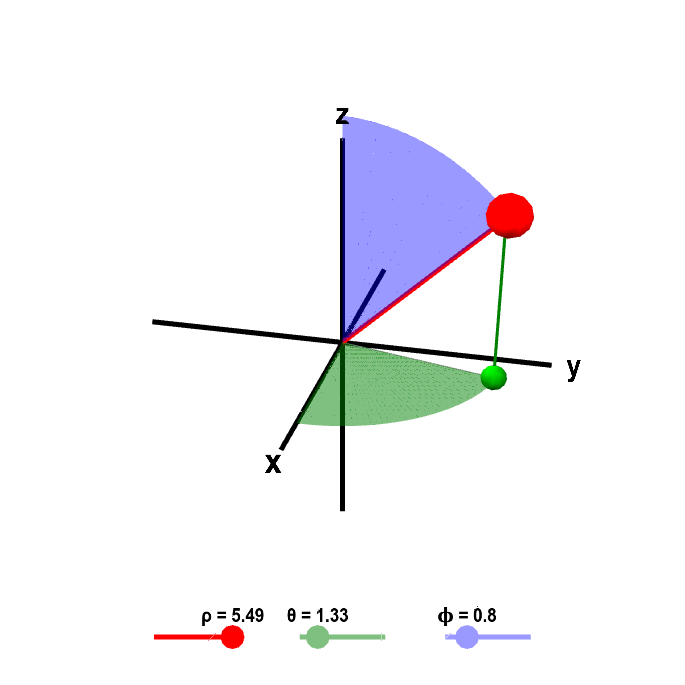# Math Insight

### Applet: Spherical coordinatesGiven the values for spherical coordinates $\rho$, $\theta$, and $\phi$, which you can change by dragging the points on the sliders, the large red point shows the corresponding position in Cartesian coordinates. The green dot is the projection of the point in the $xy$-plane. You can visualize each of the spherical coordinates by the geometric structures that are colored corresponding to the slider colors. The length of the red line segment from the origin is $\rho$. The angle of the green portion of the disk in the $xy$-plane is $\theta$. The angle of the blue portion of the vertical disk is $\phi$. You can also move the large red point and the green projection of that point directly with the mouse.